### Home > INT1 > Chapter Ch11 > Lesson 11.2.6 > Problem11-133

11-133.
1. Solve the following equations as indicated. Check your solutions if possible. Homework Help ✎

1. Solve for x: 0 =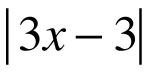2. Solve for x: x2 − 3(2x + 3) = x2 − 3

3. Solve for p: 0 = (p − 3)2p2

4. Solve for w: u =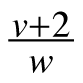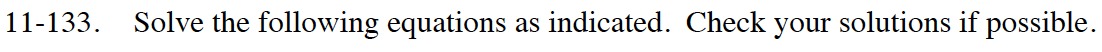x = 1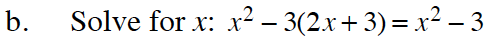Subtract x2 from both sides of the equation. Be careful to distribute a −3 over the quantity in parentheses.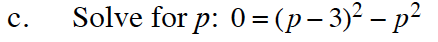(p − 3)2 = (p − 3)(p − 3)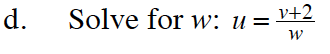Multiply both sides of the equation by w then divide both sides by u.